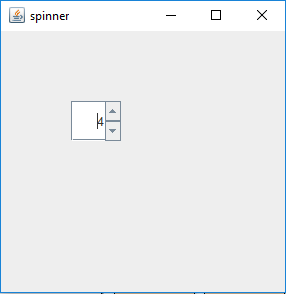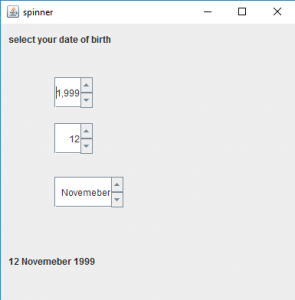# Java Swing | JSpinner

JSpinner is a part of javax.swing package . JSpinner contains a single line of input which might be a number or a object from a ordered sequence . The user can manually type in a legal data into the text field of the spinner . The spinner is sometimes preferred because they do not need a drop down list . Spinners contains a upward and a downward arrow to show the previous and the next element when it is pressed.

Constructors of JSpinner are:

1. JSpinner() : Creates an empty spinner with initial value set to zero and no contraints
2. JSpinner( SpinnerModel model)
creates a spinner with a specified spinner model passed as argument .
3. Commonly used methods are :

1. SpinnerListModel(List l) : creates a spinner model with elements of list l . This spinner model can be used to set as a model for spinner.
2. SpinnerNumberModel(int value, int max, int min, int step) : returns a spinner model whose initial value is set to value, with minimum and maximum value, and a definite step value.
3. setValue(Object v) : sets the value of the spinner to the object passed as argument.
4. getValue() : returns the current value of the spinner.
5. getPreviousValue() : returns the previous value of the spinner.
6. getNextValue() : returns the next value of the spinner.

1. Program to create a simple JSpinner

 `// java Program to create a ` `// simple JSpinner ` `import` `java.awt.event.*; ` `import` `javax.swing.*; ` `import` `java.awt.*; ` `class` `spinner ``extends` `JFrame { ` `    ``// frame ` `    ``static` `JFrame f; ` ` `  `    ``// default constructor ` `    ``spinner() ` `    ``{ ` `    ``} ` ` `  `    ``// main class ` `    ``public` `static` `void` `main(String[] args) ` `    ``{ ` `        ``// create  a new frame ` `        ``f = ``new` `JFrame(``"spinner"``); ` ` `  `        ``// create a JSpinner ` `        ``JSpinner s = ``new` `JSpinner(); ` ` `  `        ``// set Bounds for spinner ` `        ``s.setBounds(``70``, ``70``, ``50``, ``40``); ` ` `  `        ``// set layout for frame ` `        ``f.setLayout(``null``); ` ` `  `        ``// add panel to frame ` `        ``f.add(s); ` ` `  `        ``// set frame size ` `        ``f.setSize(``300``, ``300``); ` ` `  `        ``f.show(); ` `    ``} ` `} `

Output :2. Program to create a JSpinner and add ChangeListener to it ; Program to select your date of birth using JSpinner)

 `// Java program to select your ` `// date of birth using JSpinner ` `import` `java.awt.event.*; ` `import` `javax.swing.*; ` `import` `java.awt.*; ` `import` `javax.swing.event.*; ` `class` `spinner1 ``extends` `JFrame ``implements` `ChangeListener { ` `    ``// frame ` `    ``static` `JFrame f; ` ` `  `    ``// label ` `    ``static` `JLabel l, l1; ` ` `  `    ``// spinner ` `    ``static` `JSpinner s, s1, s2; ` ` `  `    ``// default constructor ` `    ``spinner1() ` `    ``{ ` `    ``} ` ` `  `    ``// main class ` `    ``public` `static` `void` `main(String[] args) ` `    ``{ ` `        ``// create an object of the class ` `        ``spinner1 sp1 = ``new` `spinner1(); ` ` `  `        ``// create  a new frame ` `        ``f = ``new` `JFrame(``"spinner"``); ` ` `  `        ``// create a label ` `        ``l = ``new` `JLabel(``"select your date of birth"``); ` `        ``l1 = ``new` `JLabel(``"1 January 2000"``); ` ` `  `        ``// create a JSpinner with a minimum, maximum and step value ` `        ``s = ``new` `JSpinner(); ` `        ``s1 = ``new` `JSpinner(``new` `SpinnerNumberModel(``1``, ``1``, ``31``, ``1``)); ` ` `  `        ``// setvalue of year ` `        ``s.setValue(``2000``); ` ` `  `        ``// store the months ` `        ``String months[] = { ``"January"``, ``"February"``, ``"March"``, ` `        ``"April"``, ``"May"``, ``"June"``, ``"July"``, ``"August"``,  ` `        ``"September"``, ``"October"``, ``"Novemeber"``, ``"December"` `}; ` ` `  `        ``// create a JSpinner with list values ` `        ``s2 = ``new` `JSpinner(``new` `SpinnerListModel(months)); ` ` `  `        ``// add change listener to spinner ` `        ``s.addChangeListener(sp1); ` `        ``s1.addChangeListener(sp1); ` `        ``s2.addChangeListener(sp1); ` ` `  `        ``// set Bounds for spinner ` `        ``s.setBounds(``70``, ``70``, ``50``, ``40``); ` `        ``s1.setBounds(``70``, ``130``, ``50``, ``40``); ` `        ``s2.setBounds(``70``, ``200``, ``90``, ``40``); ` ` `  `        ``// setbounds for label ` `        ``l.setBounds(``10``, ``10``, ``150``, ``20``); ` `        ``l1.setBounds(``10``, ``300``, ``150``, ``20``); ` ` `  `        ``// set layout for frame ` `        ``f.setLayout(``null``); ` ` `  `        ``// add label ` `        ``f.add(l); ` `        ``f.add(l1); ` `        ``f.add(s); ` `        ``f.add(s1); ` `        ``f.add(s2); ` ` `  `        ``// add panel to frame ` `        ``f.add(s); ` ` `  `        ``// set frame size ` `        ``f.setSize(``400``, ``400``); ` ` `  `        ``f.show(); ` `    ``} ` ` `  `    ``// if the state is changed ` `    ``public` `void` `stateChanged(ChangeEvent e) ` `    ``{ ` `        ``l1.setText(s1.getValue() + ``" "` `+ s2.getValue() + ``" "` `+ s.getValue()); ` `    ``} ` `} `

Output :Note : This program will not run in an online compiler please use an Offline IDE

My Personal Notes arrow_drop_upCheck out this Author's contributed articles.

If you like GeeksforGeeks and would like to contribute, you can also write an article using contribute.geeksforgeeks.org or mail your article to contribute@geeksforgeeks.org. See your article appearing on the GeeksforGeeks main page and help other Geeks.

Please Improve this article if you find anything incorrect by clicking on the "Improve Article" button below.